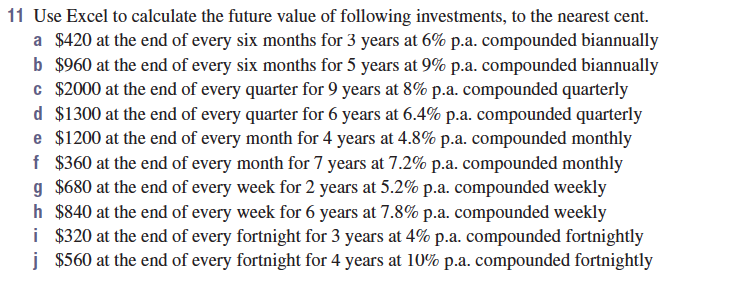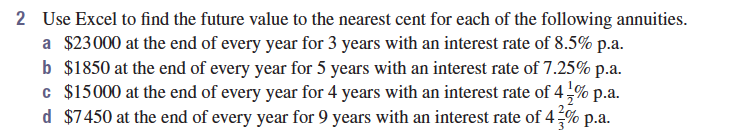# Mr Job's Maths Class

## Lessons

### Annuities

Topics:

#### Part 1 Future value of an annuity using Excel

Use Excel to create a spreadsheet as follows:

A B C D E
1 Future value of an annuity
2 Contribution: \$1000 per month
3 Annual rate: 5% Monthly rate: `=B3/12`
4 Period Present Value (PV) Interest (I) Contribution (C) P+I+C
5 1 \$0 `=B5*\$D\$3` `=\$B\$2` `=B5+C5+D5`
6

Fill row 5 down for 24 periods.

Use this spreadsheet to complete Exercise 7B, Question 11 a, c, e ...#### Part 2 Annuity calculator

Use this Annuity Calculator to complete Exercise 7B, Question 2.#### Part 3 Loan repayment calculator

Using the Loan Repayment Calculator, investigate the following:

For a loan of \$500 000,

1. How much interest is payable on this loan, with a monthly payment frequency for an interest rate of 3.5%?
2. How much less interest is payable if the payment frequency is fortnightly?

#### Part 4 Extra Repayments Calculator

Using the Extra Repayments Calculator, investigate the following:

Set the loan amount to \$500 000, the payment frequency to monthly and the interest rate to 3.5%.

Set the extra contribution to \$500 to start after 5 years.

How much interest is saved? How much time is saved off the length of the loan?

What extra contribution is required to reduce the loan length by 10 years?

Wolfram Alpha can do many maths questions.

Scientific Calculator